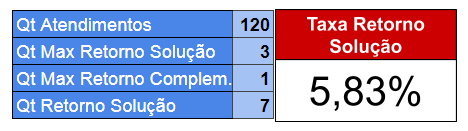# How to get specific value from a custom field?

I am using Jira Cloud I need to build a table with this information.But I can’t get the specific value of the field, it is already calculated and that’s not what I want, I need to get the maximum value of the field, but without it being added.
Only when I put to see all the lines can I see the values, but this is not what I want, I want to bring the maximum of these values ​​and mount identical to image.!
Would anyone know how to help me?

There is a function MAX you can consider for this calculation.

`Max( Set_Expression , Numeric_Expression )`

The function works over some set (any dimensions members, or measures). You would like to set some numeric value related to the set, and the MAX function will give the maximum numeric value from a set of members.

Here are two examples of MAX function used in our demo account:

1. Max resolution days in Issue Resolution Time (Boxplot Chart) report.
In this example, the formula uses a set of issues resolved in period and retrieves the maximum days it took to resolve an issue from issue creation date till resolution date.

2. Retrieve the latest date (max timestamp) to check how far to plot Time in version for Version Report.

Here I retrieved this part from the calculated measure Time in version. This example works on a set of measures and will give you a measure with the maximum value for any row:

``````TimestampToDate(Max(
{[Measures].[Version release date],
[Measures].[Predicted completion date]
},
DateToTimestamp([Measures].CurrentMember)
))
``````

Daina / support@eazybi.com